# Introduction

The purpose of this vignette is to introduce the bdpsurvival function. bdpsurvival is used for estimating posterior samples in the context of right-censored data for clinical trials where an informative prior is used. The underlying model is a piecewise exponential model that assumes a constant hazard rate for each of several sub-intervals of the time to follow-up. In the parlance of clinical trials, the informative prior is derived from historical data. The weight given to the historical data is determined using what we refer to as a discount function. There are three steps in carrying out estimation:

1. Estimation of the historical data weight, denoted $$\hat{\alpha}$$, via the discount function

2. Estimation of the posterior distribution of the current data, conditional on the historical data weighted by $$\hat{\alpha}$$

3. If a two-arm clinical trial, estimation of the posterior treatment effect, i.e., treatment versus control

Throughout this vignette, we use the terms current, historical, treatment, and control. These terms are used because the model was envisioned in the context of clinical trials where historical data may be present. Because of this terminology, there are 4 potential sources of data:

1. Current treatment data: treatment data from a current study

2. Current control data: control (or other treatment) data from a current study

3. Historical treatment data: treatment data from a previous study

4. Historical control data: control (or other treatment) data from a previous study

If only treatment data is input, the function considers the analysis a one-arm trial. If treatment data + control data is input, then it is considered a two-arm trial.

## Piecewise Exponential Model Background

Before we get into our estimation scheme, we will briefly describe the piecewise exponential model. First, we partition the time duration into $$J$$ intervals with cutpoints (or breaks) $$0=\tau_0<\tau_1<\dots<\tau_J=\infty$$. The $$j$$th interval is defined as $$[\tau_{j-1},\,\tau_j)$$. Then, we let $$\lambda_j$$ denote the hazard rate of the $$j$$th interval. That is, we assume that the hazard rate is piecewise constant.

Now, let $$d_{ij}$$ be an event indicator for the $$i$$th subject in the $$j$$th interval. That is, $$d_{ij}=1$$ if the endpoint occurred in the $$j$$th interval, otherwise $$d_{ij}=0$$. Let $$t_{ij}$$ denote the exposure time of the $$i$$th subject in the $$j$$th interval.

Let $$D_j=\sum_id_{ij}$$ be the number of episodes that occurred in interval $$j$$, and let $$T_j=\sum_it_{ij}$$ be the total exposure time within interval $$j$$. Then, the $$j$$th hazard rate is estimated as $\lambda_j\mid D_j, \sim \mathcal{G}amma\left(a_0+D_j,\,b_0+T_j\right),$ where $$a_0$$ and $$b_0$$ are the prior shape and rate parameters of a gamma distribution. The survival probability can be estimated as $p_S = 1-F_p\left(q,\,\lambda_1,\dots,\,\lambda_J,\,\tau_0,\,\dots\,\,\tau_J\right),$ where $$F_p$$ is the piecewise exponential cumulative distribution function.

In the case where a covariate effect is present, a slightly different approach is used. In the bdpsurvival function, a covariate effect arises in the context of a two-arm trial where the covariate of interest is the treatment indicator, i.e., treatment vs. control. In that case, we assume a Poisson glm model of the form $\log\mathbb{E}\left(d_{ij}\mid\lambda_j,\,\beta\right)=\log t_{ij} + \log\lambda_j + \beta I(treatment_i),\,\,\,i=1,\dots,\,N,\,\,j=1,\dots,\,J,$ where $$I(treatment_i)$$ is a treatment indicator for the $$i$$th subject. In this context, $$\beta$$ is the $$\log$$ hazard rate between the treatment and control arms. With the Poisson glm, we use an approximation to estimate $$\beta$$ conditional on each $$\lambda_j$$. Suppose we estimate hazard rates for the treatment and controls arms independently, denoted $$\lambda_{jT}$$ and $$\lambda_{jC}$$, respectively. That is $\lambda_{jT} \sim \mathcal{G}amma\left(a_0+D_{jT},\,b_0+T_{jT}\right)$ and $\lambda_{jC} \sim \mathcal{G}amma\left(a_0+D_{jC},\,b_0+T_{jC}\right),$ where $$D_{jT}$$ and $$D_{jC}$$ denote the number of events occurring in interval $$j$$ for the treatment and control arms, respectively, and $$T_{jT}$$ and $$T_{jC}$$ denote the total exposures times in interval $$j$$ for the treatment and control arms, respectively. Then, an approximation of the log-hazard rate $$\beta$$ is carried out as follows: $\begin{array}{rcl} R_j & = & \log\lambda_{jT}-\log\lambda_{jC},\,\,\,j=1,\dots,\,J,\\ \\ V_j & = & \mathbb{V}ar(R_j),\,\,\,j=1,\dots,\,J,\\ \\ \beta & = & \displaystyle{\frac{\sum_jR_j/V_j}{\sum_j1/V_j}}.\\ \end{array}$ This estimate of $$\beta$$ is a normal approximation to the estimate under a Poisson glm. Currently, the variance term $$V_j$$ is estimated empirically by calculating the variance of the posterior draws. The empirical variance approximates the theoretical variance under a normal approximation of $\begin{array}{rcl} \tilde{V}_j & = & V_{jT} + V_{jC},\\ \\ V_{jT} & = & 1/D_{jT},\\ \\ V_{jC} & = & 1/D_{jC}.\\ \end{array}$

## Estimation of the historical data weight

In the first estimation step, the historical data weight $$\hat{\alpha}$$ is estimated. In the case of a two-arm trial, where both treatment and control data are available, an $$\hat{\alpha}$$ value is estimated separately for each of the treatment and control arms. Of course, historical treatment or historical control data must be present, otherwise $$\hat{\alpha}$$ is not estimated for the corresponding arm.

When historical data are available, estimation of $$\hat{\alpha}$$ is carried out as follows. Let $$d_{ij}$$ and $$t_{ij}$$ denote the the event indicator and event time or censoring time for the $$i$$th subject in the $$j$$th interval of the current data, respectively. Similarly, let $$d_{0ij}$$ and $$t_{0ij}$$ denote the the event indicator and event time or censoring time for the $$i$$th subject in the $$j$$th interval of the historical data, respectively. Let $$a_0$$ and $$b_0$$ denote the shape and rate parameters of a gamma distribution, respectively. Then, the posterior distributions of the $$j$$th piecewise hazard rates for current and historical data, under vague (flat) priors are

$\lambda_{j} \sim \mathcal{G}amma\left(a_0+D_j,\,b_0+T_j\right),$

$\lambda_{0j} \sim \mathcal{G}amma\left(a_0+D_{0j},\,b_0+T_{0j}\right)$ respectively, where $$D_j=\sum_id_{ij}$$, $$T_j=\sum_it_{ij}$$, $$D_{0j}=\sum_id_{0ij}$$, and $$T_{j0}=\sum_it_{0ij}$$. The next steps are dependent on whether a one-arm or two-arm analysis is requested.

### Estimation under a one-arm analysis

Under a one-arm analysis, the comparison of interest is the survival probability at user-specified time $$t^\ast$$. Let $\tilde{\theta} = 1-F_p\left(t^\ast,\,\lambda_1,\dots,\,\lambda_J,\,\tau_0,\,\dots\,\,\tau_J\right),$ and $\theta_0 = 1-F_p\left(t^\ast,\,\lambda_{01},\dots,\,\lambda_{0J},\,\tau_0,\,\dots\,\,\tau_J\right),$ be the posterior survival probabilities for the current and historical data, respectively. Then, we compute the posterior probability that the current survival is greater than the historical survival $$p = Pr\left(\tilde{\theta} \ne \theta_0 \mid D, T, D_0,T_0 \right)$$, where $$D$$ and $$T$$ collect $$D_1,\dots,D_J$$ and $$T_1,\dots,T_J$$, respectively.

### Estimation under a two-arm analysis

Under a two-arm analysis, the comparison of interest is the hazard ratio of current vs. historical data. We estimate the log hazard ratio $$\beta$$ as described previously and compute the posterior probability that $$\beta \ne 0$$ as $$p = Pr\left(\beta \ne 0\mid D, T, D_0, T_0\right)$$.

Finally, for a discount function, denoted $$W$$, $$\hat{\alpha}$$ is computed as $\hat{\alpha} = \alpha_{max}\cdot W\left(p, \,w\right),\,0\le p\le1,$ where $$w$$ may be one or more parameters associated with the discount function and $$\alpha_{max}$$ scales the weight $$\hat{\alpha}$$ by a user-input maximum value. More details on the discount functions are given in the discount function section below.

There are several model inputs at this first stage. First, the user can select fix_alpha=TRUE and force a fixed value of $$\hat{\alpha}$$ (at the alpha_max input), as opposed to estimation via the discount function. Next, a Monte Carlo estimation approach is used, requiring several samples from the posterior distributions. Thus, the user can input a sample size greater than or less than the default value of number_mcmc=10000. Next, the Beta rate parameters can be changed from the defaults of $$a_0=b_0=1$$ (a0 and b0 inputs).

An alternate Monte Carlo-based estimation scheme of $$\hat{\alpha}$$ has been implemented, controlled by the function input method="mc". Here, instead of treating $$\hat{\alpha}$$ as a fixed quantity, $$\hat{\alpha}$$ is treated as random. For a one-arm analysis, let $$p_1$$ denote the posterior probability. Then, $$p_1$$ is computed as

$\begin{array}{rcl} v^2_1 & = & \displaystyle{t^{\ast2}\sum_{j=1}^{J\left(t^\ast\right)}\frac{\lambda_{j}^{2}}{D_{j}}} ,\\ \\ v^2_{01} & = & \displaystyle{t^{\ast2}\sum_{j=1}^{J\left(t^\ast\right)}\frac{\lambda_{0j}^{2}}{D_{0j}}} ,\\ \\ Z_1 & = & \displaystyle{\frac{\left|\tilde{\theta}-\theta_0\right|}{\sqrt{v^2_1 + v^2_{01}}}} ,\\ \\ p_1 & = & 2\left(1-\Phi\left(Z_1\right)\right), \end{array}$

where $$\Phi\left(x\right)$$ is the $$x$$th quantile of a standard normal (i.e., the pnorm R function). Here, $$v_1^2$$ and $$v^2_{01}$$ are estimates of the variances of $$\tilde{\theta}$$ and $$\theta_0$$, respectively. Next, $$p_1$$ is used to construct $$\hat{\alpha}$$ via the discount function. Since the values $$Z_1$$ and $$p_1$$ are computed at each iteration of the Monte Carlo estimation scheme, $$\hat{\alpha}$$ is computed at each iteration of the Monte Carlo estimation scheme, resulting in a distribution of $$\hat{\alpha}$$ values.

For a two-arm analysis, let $$p_2$$ denote the posterior probability. Then, $$p_2$$ is computed as

$\begin{array}{rcl} v^2_{2j} & = & \left(a_0 + D_j\right)^{-1},\, j=1,\dots,J,\\ \\ v^2_{02j} & = & \left(a_0 + D_{0j}\right)^{-1},\, j=1,\dots,J,\\ \\ \tilde{R} & = & \displaystyle{ \left(\sum_{j=1}^J\frac{\log\lambda_j-\log\lambda_{0j} }{1/v^2_{2j} + 1/v^2_{02j}}\right) \left(\sum_{j=1}^J\frac{1}{1/v^2_{2j} + 1/v^2_{02j}}\right)^{-1} } ,\\ \\ Z_2 & = & \displaystyle{\left|\tilde{R}\right|\left(\sum_{j=1}^J\frac{1}{1/v^2_{2j} + 1/v^2_{02j}}\right)^{-1/2} } ,\\ \\ p_2 & = & 2\left(1-\Phi\left(Z_2\right)\right), \end{array}$ where $$\Phi\left(x\right)$$ is the $$x$$th quantile of a standard normal. Here, $$v^2_{2j}$$ and $$v^2_{02j}$$ are estimates of the variances of $$\log\lambda_j$$ and $$\log\lambda_{0j}$$, respectively. Next, $$p_2$$ is used to construct $$\hat{\alpha}$$ via the discount function. Since the values $$Z_2$$ and $$p_2$$ are computed at each iteration of the Monte Carlo estimation scheme, $$\hat{\alpha}$$ is computed at each iteration of the Monte Carlo estimation scheme, resulting in a distribution of $$\hat{\alpha}$$ values.

### Discount function

There are currently three discount functions implemented throughout the bayesDP package. The discount function is specified using the discount_function input with the following choices available:

1. identity (default): Identity.

2. weibull: Weibull cumulative distribution function (CDF);

3. scaledweibull: Scaled Weibull CDF;

First, the identity discount function (default) sets the discount weight $$\hat{\alpha}=p$$.

Second, the Weibull CDF has two user-specified parameters associated with it, the shape and scale. The default shape is 3 and the default scale is 0.135, each of which are controlled by the function inputs weibull_shape and weibull_scale, respectively. The form of the Weibull CDF is $W(x) = 1 - \exp\left\{- (x/w_{scale})^{w_{shape}}\right\}.$

The third discount function option is the Scaled Weibull CDF. The Scaled Weibull CDF is the Weibull CDF divided by the value of the Weibull CDF evaluated at 1, i.e., $W^{\ast}(x) = W(x)/W(1).$ Similar to the Weibull CDF, the Scaled Weibull CDF has two user-specified parameters associated with it, the shape and scale, again controlled by the function inputs weibull_shape and weibull_scale, respectively.

Using the default shape and scale inputs, each of the discount functions are shown below.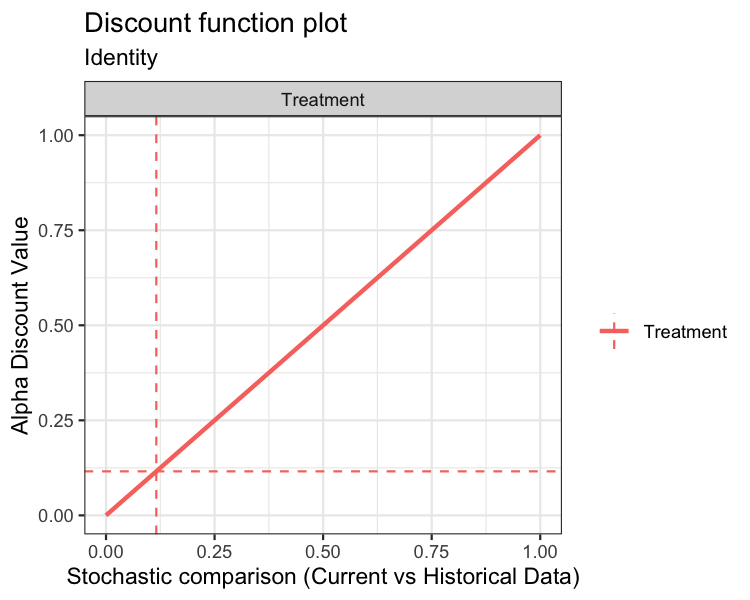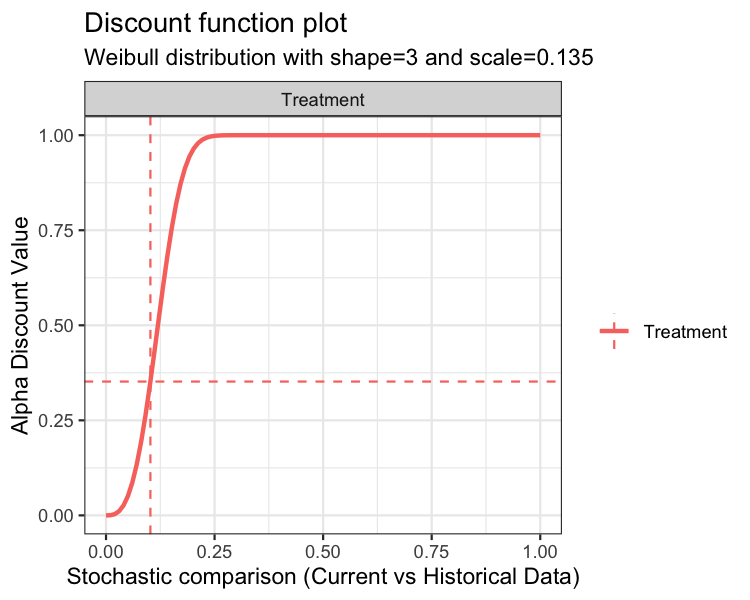In each of the above plots, the x-axis is the stochastic comparison between current and historical data, which we’ve denoted $$p$$. The y-axis is the discount value $$\hat{\alpha}$$ that corresponds to a given value of $$p$$.

An advanced input for the plot function is print. The default value is print = TRUE, which simply returns the graphics. Alternately, users can specify print = FALSE, which returns a ggplot2 object. Below is an example using the discount function plot:

p1 <- plot(fit02, type="discount", print=FALSE)
p1 + ggtitle("Discount Function Plot :-)")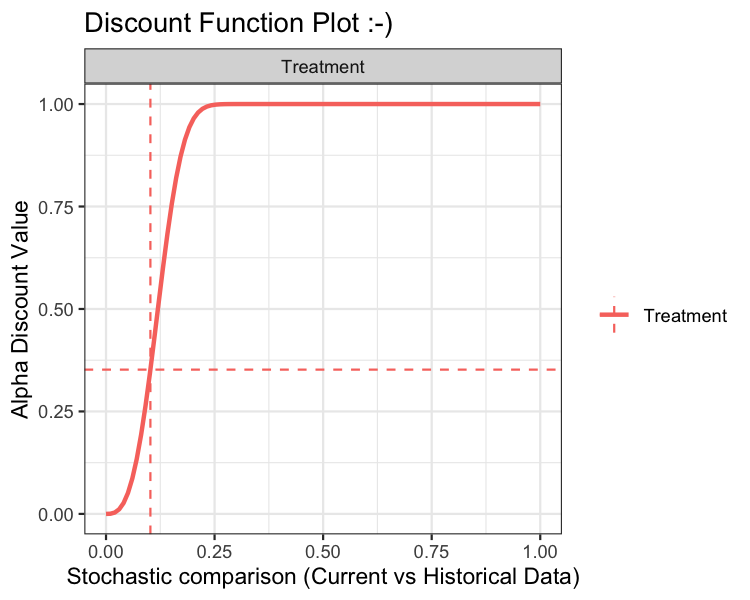## Estimation of the posterior distribution of the current data, conditional on the historical data

The posterior distribution is dependent on the analysis type: one-arm or two-arm analysis.

### Estimation under a one-arm analysis

With $$\hat{\alpha}$$ in hand, we can now estimate the posterior distributions of the hazards so that we can estimate the survival probability as described previously. Using the notation of the previous sections, the posterior distribution is $\begin{array}{rcl} p_S & = & 1-F_p\left(t^\ast,\,\lambda_1,\dots,\,\lambda_J,\,\tau_0,\,\dots\,\,\tau_J\right),\\ \\ \lambda_j & \sim & \mathcal{G}amma\left(a_0+\sum_id_{ij} + \hat{\alpha}\sum_id_{0ij},\,b_0+\sum_it_{ij} + \hat{\alpha}\sum_it_{0ij}\right),\,j=1,\dots,J\\ \end{array}$ At this model stage, we have in hand number_mcmc simulations from the augmented posterior distribution and we then generate posterior summaries.

### Estimation under a two-arm analysis

Again, under a two-arm analysis, and with $$\hat{\alpha}$$ in hand, we can now estimate the posterior distribution of the log hazard rate comparing treatment and control. Let $$\lambda_{jT}$$ and $$\lambda_{jC}$$ denote the hazard associated with the treatment and control data for the $$j$$th interval, respectively. Then we augment each of the treatment and control data by the weighted historical data as $\begin{array}{rcl} \lambda_{jT} & \sim & \mathcal{G}amma\left(a_0+\sum_id_{ijT} + \hat{\alpha}_T\sum_id_{0ijT},\,b_0+\sum_it_{ijT} + \hat{\alpha}_T\sum_it_{0ijT}\right),\,j=1,\dots,J\\ \end{array}$ and $\begin{array}{rcl} \lambda_{jC} & \sim & \mathcal{G}amma\left(a_0+\sum_id_{ijC} + \hat{\alpha}_C\sum_id_{0ijC},\,b_0+\sum_it_{ijC} + \hat{\alpha}_C\sum_it_{0ijC}\right),\,j=1,\dots,J\\ \end{array}$ respectively. We then construct the log hazard ratio $$\beta$$ using the estimates of $$\lambda_{jT}$$ and $$\lambda_{jC}$$, $$j=1,\dots,\,J$$ as described previously. At this model stage, we have in hand number_mcmc simulations from the augmented posterior distribution and we then generate posterior summaries.

## Inputting Data

Data for bdpsurvival is input via data frame(s) that must have certain columns. Though bdpsurvival supports analyses with no historical data, other packages/functions should be used.

To carry out an analysis using historical data, two data frames need to be constructed: (1) current data, input via data; and (2) historical data, input via data0. The analysis type, i.e., one-arm vs. two-arm, is controlled via the right-hand-side (RHS) of the formula input.

Suppose we have data frames with matching column names. The survival times are stored in the time column while the event indicator is stored in the status column. Then, the formula input Surv(time, status) ~ 1 specifies a one-arm treatment. As long as the term treatment does not appear on the RHS of the formula, the analysis will be one-arm.

Now, suppose we desire a two-arm analysis. Then, the current and historical data frames must contain a column named exactly treatment. The treatment column should be binary and indicates treatment and control observations. Now, the formula input Surv(time, status) ~ treatment specifies a two-arm treatment.

# Examples

## One-arm trial

To demonstrate a one-arm trial, we will simulate a small survival dataset from an exponential distribution. For ease of exposition, we will assume that there are no censored observations.

set.seed(42)
# Simulate survival times for current and historical data
surv_1arm <- data.frame(status = 1,
time   = rexp(10, rate=1/10))

# Simulate survival times for historical data
surv_1arm0 <- data.frame(status = 1,
time   = rexp(50, rate=1/11))

In this example, we’ve simulated current survival times from an exponential distribution with rate 1/10 and historical survival times from an exponential distribution with rate 1/11; status = 1 for all observations, implying no times are censored. With our data frame constructed, we can now fit the bdpsurvival model. Since this is a one-arm trial, we will request the survival probability at surv_time=5. Thus, estimation using the default model inputs is carried out:

set.seed(42)
fit1 <- bdpsurvival(Surv(time, status) ~ 1,
data  = surv_1arm,
data0 = surv_1arm0,
surv_time = 5,
method = "fixed")
print(fit1)
##
##     One-armed bdp survival
##
##
##   n events surv_time median lower 95% CI upper 95% CI
##  10     10         5 0.5259       0.3179       0.7355

The print method displays the median survival probability of 0.5259 and the 95% lower and upper interval limits of 0.3179 and 0.7355, respectively. The summary method is implemented as well. For a one-arm trial, the summary outputs a survival table for the current data as follows:

summary(fit1)
##
##     One-armed bdp survival
##
## Stochastic comparison (p_hat) - treatment (current vs. historical data): 0.188
## Discount function value (alpha) - treatment: 0.188
##
## Current treatment - augmented posterior summary:
##     time n.risk n.event survival std.err lower 95% CI upper 95% CI
##   0.3819     23       1   0.9688  0.0149       0.9323       0.9894
##   1.9834     22       1   0.8483  0.0656       0.6948       0.9464
##   2.8349     21       1   0.7905  0.0860       0.5942       0.9243
##   3.1278     20       0   0.7715  0.0921       0.5631       0.9168
##   3.1398     13       1   0.7699  0.0919       0.5619       0.9143
##   4.1013     12       1   0.6294  0.0938       0.4360       0.8006
##   4.7318     11       1   0.5551  0.1037       0.3502       0.7543
##   5.0666     10       0   0.5189  0.1088       0.3096       0.7311
##   6.6090      6       1   0.4294  0.0992       0.2425       0.6301
##   7.1486      5       1   0.4016  0.0990       0.2194       0.6074
##   9.1584      4       0   0.3165  0.1016       0.1424       0.5365
##  11.9160      2       1   0.2230  0.0823       0.0933       0.4091
##  14.6363      1       1   0.1599  0.0759       0.0513       0.3425

In the above output, in addition to a survival table, we can see the stochastic comparison between the current and historical data of 0.188 as well as the weight, alpha, of 0.188 applied to the historical data. Here, the weight applied to the historical data is low since the stochastic comparison suggests that the current and historical data are not similar.

Suppose that we would like to apply full weight to the historical data. This can be accomplished by setting alpha_max=1 and fix_alpha=TRUE as follows:

set.seed(42)
fit1a <- bdpsurvival(Surv(time, status) ~ 1,
data  = surv_1arm,
data0 = surv_1arm0,
surv_time = 5,
alpha_max = 1,
fix_alpha = TRUE,
method = "fixed")

print(fit1a)
##
##     One-armed bdp survival
##
##
##   n events surv_time median lower 95% CI upper 95% CI
##  10     10         5 0.6041       0.4762         0.72

Now, the median survival probability shifts upwards towards the historical data.

Many of the the values presented in the summary and print methods are accessible from the fit object. For instance, alpha is found in fit1a$posterior_treatment$alpha_discount and p_hat is located at fit1a$posterior_treatment$p_hat. The augmented survival probability and CI are computed at run-time. The results can be replicated as:

survival_time_posterior <- ppexp(5,
fit1a$posterior_treatment$posterior_hazard,
cuts=c(0,fit1a$args1$breaks))
surv_augmented <- 1-median(survival_time_posterior)
CI95_augmented <- 1-quantile(survival_time_posterior, prob=c(0.975, 0.025))

Here, we first compute the piecewise exponential cumulative distribution function using the ppexp function. The ppexp function requires the survival time, 5 here, the posterior draws of the piecewise hazards, and the cuts points of the corresponding intervals.

Finally, we’ll explore the plot method.

plot(fit1, type="survival")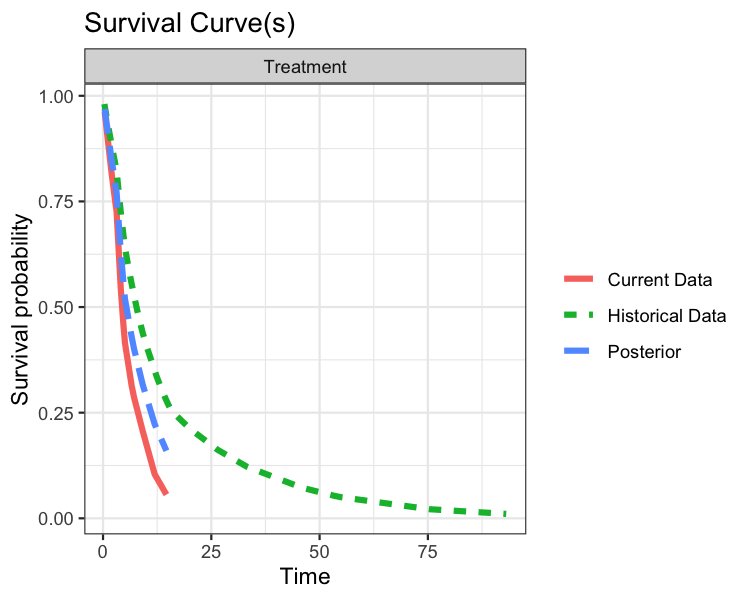plot(fit1, type="discount")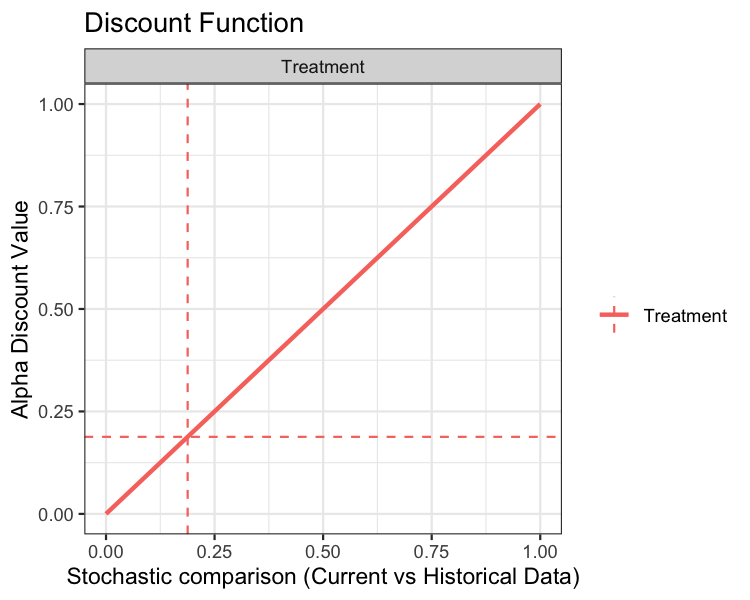The top plot displays three survival curves. The green curve is the survival of the historical data, the red curve is the survival of the current event rate, and the blue curve is the survival of the current data augmented by historical data. Since we gave full weight to the historical data, the augmented curve is “in-between” the current and historical curves.

The bottom plot displays the discount function (solid curve) as well as alpha (horizontal dashed line) and p_hat (vertical dashed line). In the present example, the discount function is the identity.

## Two-arm trial

On to two-arm trials. In this package, we define a two-arm trial as an analysis where a current and/or historical control arm is present. Suppose we have the same treatment data as in the one-arm example, but now we introduce control data. Again, we will assume that there is no censoring present in the control data:

set.seed(42)
# Simulate survival times for treatment data
time_current_trt    <- rexp(10, rate=1/10)
time_historical_trt <- rexp(50, rate=1/11)

# Simulate survival times for control data
time_current_cntrl    <- rexp(10, rate=1/12)
time_historical_cntrl <- rexp(50, rate=1/12)

# Combine simulated data into data frames
surv_2arm <- data.frame(treatment = c(rep(1,10),rep(0,10)),
time      = c(time_current_trt, time_current_cntrl),
status    = 1)

surv_2arm0 <- data.frame(treatment = c(rep(1,50),rep(0,50)),
time      = c(time_historical_trt, time_historical_cntrl),
status    = 1)

In this example, we’ve simulated current and historical control survival times from exponential distributions with rate 1/12. Note how the data frames have been constructed; we’ve taken care to ensure that the current/historical and treatment/control indicators line up properly. With our data frame constructed, we can now fit the bdpsurvival model. Before proceeding, it is worth pointing out that the discount function is applied separately to the treatment and control data. Now, let’s carry out the two-arm analysis using default inputs:

set.seed(42)
fit2 <- bdpsurvival(Surv(time, status) ~ treatment,
data = surv_2arm,
data0 = surv_2arm0,
method = "fixed")
print(fit2)
##
##     Two-armed bdp survival
##
## data:
##   Current treatment: n = 23, number of events = 10
##   Current control: n = 24, number of events = 10
## Stochastic comparison (p_hat) - treatment (current vs. historical data): 0.1264
## Stochastic comparison (p_hat) - control (current vs. historical data): 0.0618
## Discount function value (alpha) - treatment: 0.1264
## Discount function value (alpha) - control: 0.0618
##
##             coef exp(coef) se(coef) lower 95% CI upper 95% CI
## treatment -0.151    0.8599   0.4122      -0.9542       0.6606

The print method of a two-arm analysis is largely different than a one-arm analysis (with a two-arm analysis, the summary method is identical to print). First, we see the stochastic comparisons reported for both the treatment and control arms. As seen previously, the stochastic comparison between the current and historical data for the treatment data is relatively small at 0.1264, giving a low historical data weight of 0.1264. Similarly, the stochastic comparison between the current and historical data for the control data is relatively low at 0.0618, giving a low historical data weight of 0.0618. Finally, the presented coef value (and associated interval limits) is the log hazard ratio between the augmented treatment data and the augmented control data computed as log(treatment) - log(control).